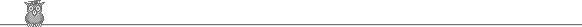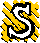### 28th Putnam 1967A1.  We are given a positive integer n and real numbers ai such that |∑1n ak sin kx| ≤ |sin x| for all real x. Prove |∑1n k ak| ≤ 1.A2.  Let un be the number of symmetric n x n matrices whose elements are all 0 or 1, with exactly one 1 in each row. Take u0 = 1. Prove un+1 = un + n un-1 and ∑0∞ un xn/n! = ef(x), where f(x) = x + (1/2) x2.A3.  Find the smallest positive integer n such that we can find a polynomial nx2 + ax + b with integer coefficients and two distinct roots in the interval (0, 1).A4.  Let 1/2 < α ∈ R, the reals. Show that there is no function f : [0, 1] → R such that f(x) = 1 + α ∫x1 f(t) f(t - x) dt for all x ∈ [0, 1].A5.  K is a convex, finite or infinite, region of the plane, whose boundary is a union of a finite number of straight line segments. Its area is at least π/4. Show that we can find points P, Q in K such that PQ = 1.A6.  ai and bi are reals such that a1b2 ≠ a2b1. What is the maximum number of possible 4-tuples (sign x1, sign x2, sign x3, sign x4) for which all xi are non-zero and xi is a simultaneous solution of a1x1 + a2x2 + a3x3 + a4x4 = 0 and b1x1 + b2x2 + b3x3 + b4x4 = 0. Find necessary and sufficient conditions on ai and bi for this maximum to be achieved.B1.  A hexagon is inscribed in a circle radius 1. Alternate sides have length 1. Show that the midpoints of the other three sides form an equilateral triangle.B2.  α, β ∈ [0, 1] and we have ax2 + bxy + cy2 ≡ (αx + (1 - α)y)2, (αx + (1 - α)y)(βx + (1 - β)y) ≡ dx2 + exy + fy2. Show that at least one of a, b, c ≥ 4/9 and at least one of d, e, f ≥ 4/9.B3.  R is the reals. f, g are continuous functions R → R with period 1. Show that limn→∞ ∫01 f(x) g(nx) dx = (∫01 f(x) dx) (∫01 g(x) dx).B4.  We are given a sequence a1, a2, ... , an. Each ai can take the values 0 or 1. Initially, all ai = 0. We now successively carry out steps 1, 2, ... , n. At step m we change the value of ai for those i which are a multiple of m. Show that after step n, ai = 1 iff i is a square. Devise a similar scheme to give ai = 1 iff i is twice a square.B5.  The first n terms of the exansion of (2 - 1)-n are 2-n ( 1 + n/1! (1/2) + n(n + 1)/2! (1/2)2 + ... + n(n + 1) ... (2n -2)/(n - 1)! (1/2)n-1 ). Show that they sum to 1/2.B6.  R is the reals. D is the closed unit disk x2 + y2 ≤ 1 in R2. The function f : D → [-1, 1] has partial derivatives f1(x, y) and f2(x, y). Show that there is a point (a, b) in the interior of D such that f1(a, b)2 + f2(a, b)2 ≤ 16.To avoid possible copyright problems, I have changed the wording, but not the substance, of all the problems. The original text and official solutions were published in American Mathematical Monthly 75 (1968) 734-9. They are also available (with the solutions expanded) in: Gerald L Alexanderson et al, The William Lowell Putnam Mathematical Competition, 1965-1984. Out of print, but in some university libraries.

Putnam home